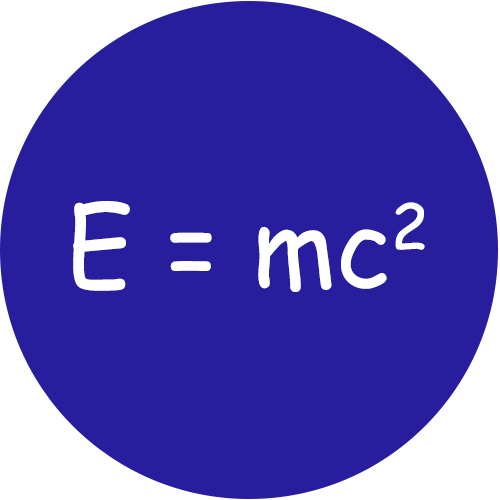# Mass to Energy Calculator

Use our Mass to Energy Calculator a free online tool to calculate the equivalence value for the given inputs.Enter the values of mass and speed of light in a vacuum to calculate.

Energy(E)=m * c2

The unit of Energy is Joules(J)